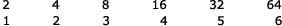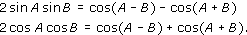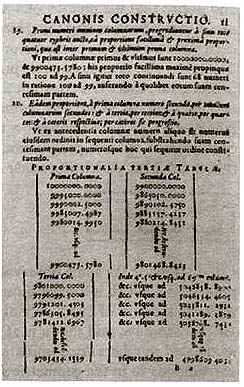John Napier

Start this free course now. Just create an account and sign in. Enrol and complete the course for a free statement of participation or digital badge if available.

Free course

# 1.3 Napier's approach to logarithms

Napier's major and more lasting invention, that of logarithms, forms a very interesting case study in mathematical development. Within a century or so what started life as merely an aid to calculation, a set of ‘excellent briefe rules’, as Napier called them, came to occupy a central role within the body of theoretical mathematics.

The basic idea of what logarithms were to achieve is straightforward: to replace the wearisome task of multiplying two numbers by the simpler task of adding together two other numbers. To each number there was to be associated another, which Napier called at first an ‘artificial number’ and later a ‘logarithm’ (a term which he coined from Greek words meaning something like ‘ratio-number’), with the property that from the sum of two such logarithms the result of multiplying the two original numbers could be recovered.

In a sense this idea had been around for a long time. Since at least Greek times it had been known that multiplication of terms in a geometric progression could correspond to addition of terms in an arithmetic progression. For instance, considerand notice that the product of 4 and 8 in the top line, viz 32, lies above the sum of 2 and 3 in the bottom line (5). (Here the top line is a geometric progression, because each term is twice its predecessor; there is a constant ratio between successive terms. The lower line is an arithmetic progression, because each term is one more than its predecessor; there is a constant difference between successive terms.) Precisely these two lines appear as parallel columns of numbers on an Old Babylonian tablet, though we do not know the scribe's intention in writing them down.

A continuation of these progressions is the subject of a passage in Chuquet's Triparty (1484). Read the passage, linked below, now.

Click the link below to open the passage from Chuquet's Triparty.

Nicolas Chuquet on exponents [Tip: hold Ctrl and click a link to open it in a new tab. (Hide tip)]

Chuquet made the same observation as above, that the product of 4 and 8 (‘whoever multiplies 4 which is a second number by 8 which is a third number’) gives 32, which is above the sum of 2 and 3 (‘makes 32 which is a fifth number’). Chuquet seems virtually to have said that a neat way of multiplying 4 and 8 is to add their associated numbers (‘denominations’) in the arithmetic series and see what the result corresponds to in the geometric series. (Of course, had he wanted to multiply 5 by 9, say, Chuquet would have been stuck.) And in The sand-reckoner, long before, Archimedes proved a similar result for any geometric progression.

So the idea that addition in an arithmetic series parallels multiplication in a geometric one was not completely unfamiliar. Nor, indeed, was the notion of reaching the result of a multiplication by means of an addition. For this was quite explicit in trigonometric formulae discovered early in the sixteenth century, such as:Thus if you wanted to multiply two sines, or two cosines, together – a very nasty calculation on endlessly fiddly numbers – you could reach the answer through the vastly simpler operation of subtracting or adding two other numbers. This method was much used by astronomers towards the end of the sixteenth century, particularly by the great Danish astronomer Tycho Brahe, who was visited by a young friend of Napier, John Craig, in 1590. So Napier was probably aware of these techniques at about the time he started serious work on his own idea, although conceptually it was entirely different.

Napier's definition of logarithm is rather interesting. We shall not pursue all its details, but just enough to see its approach and character. Imagine two points, P and L, each moving along its own line.Figure 3

The line P0 Q is of fixed, finite length, but L's line is endless. L travels along its line at constant speed, but P is slowing down. P and L start (from P0 and L0) with the same speed, but thereafter P's speed drops proportionally to the distance it has still to go: at the half-way point between P0 and Q, P is travelling at half the speed they both started with; at the three-quarter point, it is travelling with a quarter of the speed; and so on. So P is never actually going to get to Q, any more than L will arrive at the end of its line, and at any instant the positions of P and L uniquely correspond. Then at any instant the distance L0L is, in Napier's definition, the logarithm of the distance PQ. (That is, the numbers measuring those distances.) Thus the distance L has travelled at any instant is the logarithm of the distance P has yet to go.

How does this cohere with the ideas we spoke of earlier? The point L moves in an arithmetic progression: there is a constant difference between the distance it moves in equal time intervals – that is what ‘constant speed’ means. The point P, however, is slowing down in a geometric progression: its motion was defined so that it was the ratio of successive distances that remained constant in equal time intervals.Figure 4 From Napier's Constructio (1619). Notice that the seven significant figures afforded by the whole sine of 107 are not sufficient for the accuracy he desired, and he used four more, beyond the ‘decimal point’ – one of the earliest occurrences of our decimal point symbol in print, which helped to stabilise this notation in its now-familiar form

## Question 2

Compare what you have gleaned of Napier's concept of logarithm with earlier ideas about progressions and trigonometrical formulae. What seems to you the most striking difference in overall approach? What problem do you see Napier's work solving?

### Discussion

The major difference is surely in his use of the concept of motion, of points moving along lines with speeds defined in various ways. Both exponents of Chuquet's kind and trigonometrical formulae are quite ‘static’ objects by comparison – there is evidently a deep difference of mathematical style here.

What seems so clever about Napier's approach is that he can cope with any number, in effect, not just the ones that happen to form part of some particular discrete geometrical progression. This is effected by his intuition springing from the continuous nature of the straight line and of motion.

MA290_3

### Take your learning further

Making the decision to study can be a big step, which is why you'll want a trusted University. The Open University has 50 years’ experience delivering flexible learning and 170,000 students are studying with us right now. Take a look at all Open University courses.

If you are new to University-level study, we offer two introductory routes to our qualifications. You could either choose to start with an Access module, or a module which allows you to count your previous learning towards an Open University qualification. Read our guide on Where to take your learning next for more information.

Not ready for formal University study? Then browse over 1000 free courses on OpenLearn and sign up to our newsletter to hear about new free courses as they are released.

Every year, thousands of students decide to study with The Open University. With over 120 qualifications, we’ve got the right course for you.

Request an Open University prospectus371